## research papersJOURNAL OFSYNCHROTRONRADIATION
ISSN: 1600-5775
Volume 25| Part 6| November 2018| Pages 1797-1802

## Experimental feasibility of dual-energy computed tomography based on the Thomson scattering X-ray source

aDepartment of Engineering Physics, Tsinghua University, Beijing 100084, People's Republic of China, and bCollege of Nuclear Science and Technology, Beijing Normal University, Beijing 100875, People's Republic of China
*Correspondence e-mail: dych@mail.tsinghua.edu.cn

(Received 14 July 2018; accepted 6 September 2018; online 28 September 2018)

Unlike large-scale and expensive synchrotron radiation facilities, the Thomson scattering X-ray source can provide quasi-monochromatic, energy-tunable and high-brightness X-ray pulses with a small footprint and moderate cost, making it an excellent candidate for dual-energy and multi-energy imaging at laboratories and hospitals. Here, the first feasibility study on dual-energy computed tomography (CT) based on this type of light source is reported, and the effective atomic number and electron-density distribution of a standard phantom consisting of polytetrafluoroethylene, water and aluminium is derived. The experiment was carried out at the Tsinghua Thomson scattering X-ray source with peak energies of 29 keV and 68 keV. Both the reconstructed effective atomic numbers and the retrieved electron densities of the three materials were compared with their theoretical values. It was found that these values were in agreement by 0.68% and 2.60% on average for effective atomic number and electron density, respectively. These results have verified the feasibility of dual-energy CT based on the Thomson scattering X-ray source and will further expand the scope of X-ray imaging using this type of light source.

### 1. Introduction

Since the invention of the first clinical instrument in the early 1970s, X-ray computed tomography (CT) has been developed into an indispensable tool for medical diagnostics and research. However, there are still limitations of routine single-energy CT examination in practical applications. For example, various tumors (e.g. liver, head and oral cancer) can hardly be delineated as a result of their low contrasts in CT imaging; it is also difficult to differentiate uric acid from calcium in musculoskeletal tissue in tophaceous gout diagnostics because of their similar attenuations. With the development of dual-energy CT, the above tumor delineation (Agrawal et al., 2014; Tawfik et al., 2012; Forghani & Mukherji, 2018; Toepker et al., 2014) and tissue component differentiation (Desai et al., 2011) can be effectively resolved. Furthermore, the effective atomic number Zeff and electron density ρe can also be obtained using a dual-energy CT scan, which plays an important role in the precise dose calculation (Bazalova et al., 2008; Landry et al., 2011; van Elmpt et al., 2016) and range estimation (Yang et al., 2010; Hünemohr et al., 2013, 2014; Hudobivnik et al., 2016) in proton- and ion-therapy treatment planning.

In terms of dual-energy CT, both the early rapid tube potential switching (Kalender et al., 1986), layered detector (Ishigaki et al., 1988; Stewart & Huang, 1990) techniques and the recently developed dual-source scheme (Flohr et al., 2006; Johnson et al., 2007) are suffering from the broad-spectrum characteristics of conventional X-ray tubes, meaning the reconstruction precision is still limited. The advent of the photon-counting detector (Schlomka et al., 2008; Shikhaliev, 2008) provides a more robust solution to dual-energy CT and also opens the door to multi-energy CT. This technology, however, is still immature and suffers from severe count-rate and pixel-size limitations that are critical for high-speed and high-resolution imaging (Taguchi & Iwanczyk, 2013). The new proposed transXend detector (Kanno et al., 2008; Yamashita et al., 2014) also provides a way for energy-resolved CT by measuring the electric current to avoid pile-up problems at high X-ray flux, although its energy resolution is not excellent. From a source point of view, synchrotron radiation is very suitable for dual-energy CT. Based on its highly monochromatic X-rays, high dual-energy reconstruction precisions of Zeff and ρe have been experimentally verified using different samples (Torikoshi et al., 2003; Tsunoo et al., 2004). However, the large-scale footprint and expense of a synchrotron facility limits its application in fundamental research.

As an alternative, the Thomson scattering X-ray source, also known as the inverse Compton scattering X-ray source, provides valuable prospects for dual-energy CT, since it can provide quasi-monochromatic, energy-tunable and high-brightness X-rays with a small footprint and relatively low costs. Besides, the scattered X-rays also have other excellent qualities, such as high spatial coherence, precisely manipulated polarization and ultra-short pulse length. Hence, there are many important applications based on this type of light source, including monochromatic CT (Achterhold et al., 2013; Chi et al., 2017a,b), energy-resolved CT, phase-contrast imaging (Ikeura-Sekiguchi et al., 2008; Oliva et al., 2010; Bech et al., 2009; Schleede et al., 2012; Zhang et al., 2014; Eggl et al., 2015; Chi et al., 2017c), radiation therapy (Jacquet & Suortti, 2015), protein crystallography (Hartemann et al., 2001) and ultrafast process diagnostics (Schoenlein et al., 1996).

In this paper, we demonstrate the first experimental feasibility of dual-energy CT based on the Thomson scattering X-ray source and present the dual-energy imaging results obtained at the Tsinghua Thomson scattering X-ray source (TTX).

### 2. Materials and methods

#### 2.1. The TTX beamline

As the first hard X-ray source based on Thomson scattering in China, the TTX aims to generate high-brightness monochromatic X-rays ranging from 20 keV to 50 keV (Du et al., 2013). After a series of upgrades, the TTX can now produce X-ray pulses in the energy range 20–70 keV, and the total photon yield has increased to 2 × 107 photons per pulse. A schematic layout of the TTX beamline is shown in Fig. 1(a). Electron bunches are generated through the photocathode RF gun whose Cu cathode is illuminated by the UV laser (266 nm) at a repetition of 10 Hz. The electron bunch is then accelerated to relativistic energies (30–54 MeV) in a 3 m S-band linac and collides with the infrared laser pulse (800 nm) at a head-on geometry after being focused and matched.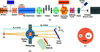Figure 1 (a) Schematic layout of the TTX beamline, not to scale. (b) Experimental layout of the dual-energy CT measurement. (c) Structure of the phantom.

When the dual-energy CT experiment was carried out, the beam parameters at the interaction point (IP) (outlined in Fig. 1a) were as follows: for the electron bunch, energies of 35 MeV and 53.5 MeV, 400 pC bunch charge, a normalized r.m.s. emittance of ∼1.5 mm mrad, ∼60 µm r.m.s. spot size and an r.m.s. energy spread of ∼0.3%; for the laser beam, a pulse energy of 200 mJ, an r.m.s. waist size of ∼3 µm and an r.m.s. bandwidth of ∼2.2%. Based on the above beam parameters, the X-ray peak energy Ex can be calculated according to the relation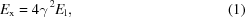yielding low and high energies of 29 keV and 68 keV. In equation (1), γ and El are the relativistic Lorentz factors of the electron and laser photon energies, respectively. The intrinsic r.m.s. bandwidth of scattered X-rays is ∼2.4%, measured based on the crystal-diffraction method (Chi et al., 2017d). The divergence angle of scattered X-rays is ∼6 mrad, confined by the titanium window so that a transverse beam size of ∼3 cm can be obtained at a typical imaging distance of 2.5 m from the IP. A larger field of view can be easily obtained by enlarging the imaging distance; however, the photon flux will be reduced.

#### 2.2. Imaging system and sample

The experimental layout of dual-energy CT is shown in Fig. 1(b). A high-precision rotation stage (URS75BCC, Newport) was placed on a horizontal sliding stage 2.5 m downstream of the IP, so that the sample images and flat-field image used for intensity normalization can be easily recorded by moving the sample inside and outside of the X-ray beam. Both the flat image and sample images were acquired through an EMCCD camera (Andor) coupled with a Lanex screen at 0.6 m downstream from the sample. When the incident X-rays arrive at the Lanex screen, visible light is excited and then captured by the EMCCD. In order to effectively collect the visible light, the Lanex screen was placed at an angle of φ = 31.5with respect to the x axis direction (cf. Fig. 1b). This irregular detector geometry can be easily corrected through a suitable reconstruction algorithm. The spatial resolution of the imaging system is ∼150 µm after calibration.

The imaging object was a cylindrical polytetrafluoro­ethylene (PTFE) phantom (radius 5 mm), inside which there were two small cylinders made of different materials: aluminium (radius 1 mm) and water (radius 2 mm), as shown in Fig. 1(c). The H2O was used to calibrate the reconstructed pixel values of the CT images, so that the value of the linear attenuation coefficient can be directly measured from the reconstructed CT images. The small size of the sample can avoid the influence of the energy–angle correlation associated with Thomson light sources on the CT reconstruction (Chi et al., 2017a) and guarantee a relatively higher photon flux at the same time. During the CT scan, the sample was placed on the high-precision rotation stage and rotated at step intervals of 10. Owing to the relatively low repetition of the TTX (i.e. 10 Hz), the CT data acquisition is time-consuming (∼2 min per projection) to obtain relatively high signal-to-noise ratio (SNR) images. Hence, only 36 projections were taken over 360for each single-energy CT scan.

#### 2.3. Image reconstruction

Considering that the limited projection number cannot meet the requirement of the conventional filtered back-projection reconstruction algorithm, an effective algebraic reconstruction referred to as the simultaneous algebraic reconstruction technique (SART) (Andersen & Kak, 1984) was adopted. Another reason to choose the algebraic reconstruction algorithm was to correct the irregular detector arrangement (cf. Fig. 1b), since it can be easily dealt with in the system matrix of the SART algorithm. Before the CT reconstruction, a median filter was applied to the raw images to improve the image SNR.

After reconstruction of the linear attenuation coefficient distributions μ(EL, r) (r denoting a vector in Euclidean space) and μ(EH, r) at low energy EL and high energy EH, respectively, the Zeff(r) distribution can be retrieved by solving the following equation,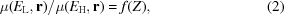where f(Z) is a monotonic function of the atomic number Z and was tabulated previously using the data from the National Institute of Standards and Technology (NIST) (see https://www.nist.gov/pml/X-ray-mass-attenuation-coefficients for details). Hence, equation (2)can be resolved using the look-up table method, and the tabulated function in our experiment is shown in Fig. 2.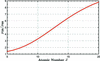Figure 2 Relationship of μ(29 keV)/μ(68 keV) versus Z from NIST data, based on which Zeff(r) can be retrieved from the dual-energy reconstructed results of the linear attenuation coefficient.

When the Zeff(r) distribution is retrieved, the ρe(r) information reconstruction is straightforward. According to the dual-energy decomposition theory of the linear attenuation coefficient [μ(E)] (Alvarez & Macovski, 1976), μ(E) of a certain material, in the keV energy regime, can be decomposed linearly into two basis functions,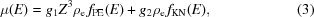where fPE(E) = 1/E3 is the energy dependence of the photoelectric effect, fKN(E) is the Klein–Nishina function that describes the energy dependence of the Compton scattering effect, and g1 and g2 approximate constants. In equation (3), we adopt the definition of electron density ρe as follows,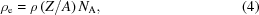with ρ, A and NA representing the mass density, atomic weight and Avogadro's constant, respectively. Based on equation (3), the ρe(r) distribution can be easily reconstructed,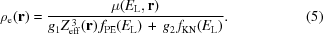### 3. Results and discussions

There were 200 slices of sample acquired along the rotation axis direction, and a typical slice of the reconstructed linear attenuation coefficient distribution at low and high energies is shown in Fig. 3. The three materials in the sample can be distinguished from each other based on the differences in their linear attenuation coefficients in both the low- and high-energy cases. In order to quantitatively analyze the reconstruction results, three regions of interest (ROIs) shown in Figs. 3(a) and 3(b) highlighted by pink dotted squares are chosen. The average of the reconstructed linear attenuation coefficient and its standard deviation over all pixels in the ROI are listed in Table 1. Also given in Table 1are the theoretical values obtained from the NIST database and the relative ratios of the measured results to the reference values. Note that excellent accuracy (<1%) is achieved for the high-attenuation material Al at 29 keV and 68 keV, whereas the reconstruction accuracy for weak attenuation material PTFE is slightly lower (4.00% at 29 keV, 6.28% at 68 keV). Since both the photon flux of the TTX and the detection efficiency of the detection system are relatively low, the SNR of the imaging system is not high. Hence, the reconstruction errors of the linear attenuation coefficients of the three materials are relatively high (≳5%, cf. the second column of Table 1). Besides, the weaker the X-ray attenuation of a material, the higher the reconstruction error obtained at the same energy, because the CT reconstruction of a material with a lower linear attenuation coefficient is more sensitive to noise. Compared with the reconstruction errors of the three materials at 29 keV, their counterparts at 68 keV are much lower, which can be attributed to the improved SNR at 68 keV resulting from the photon-flux increase.

 Table 1Linear attenuation coefficient: reconstructed values, reference values and their comparison
Sample Reconstructed value μC (cm−1) Reference value μR (cm−1) Comparison ∣μC/μR−1∣ (%)
Al (29 keV) 3.40 ± 0.21 3.40 0.13
H2O (29 keV) 0.41 ± 0.08 0.41 0.06
PTFE (29 keV) 0.95 ± 0.08 0.99 4.00
Al (68 keV) 0.66 ± 0.03 0.66 0.50
H2O (68 keV) 0.20 ± 0.02 0.20 0.04
PTFE (68 keV) 0.37 ± 0.02 0.40 6.28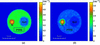Figure 3 Linear attenuation coefficient images of the sample at (a) 68 keV and (b) 29 keV. The pink dotted squares in each image are the three ROIs chosen for the quantitative analysis of the reconstructed results.

Based on the dual-energy reconstruction results of the linear attenuation coefficient, the effective atomic number Zeff and electron density ρe of the sample can be easily retrieved using the method described in §2.3, and the results are shown in Fig. 4. Since the Zeff values of H2O are very close to those of PTFE, it is therefore difficult to distinguish them from each other in the image of effective atomic number, while Al can be easily identified because of its relatively high Zeff value, as shown in Fig. 4(a). On the other hand, H2O can be easily identified among the three materials based on the electron density image because of its relatively low ρe value; however, Al is hardly distinguishable from PTFE as a result of their similar ρe values, as shown in Fig. 4(b). For quantitative analysis of the retrieved Zeff and ρe results, three ROIs chosen in the quantitative analysis of the reconstructed linear attenuation coefficient are also taken into consideration. The comparisons between reconstructed results and theoretical values are shown in Tables 2 and 3 for Zeff and ρe, respectively, and the data points against the reference values (solid lines) are plotted in Fig. 5for visual comparison. Also shown in Fig. 5are the ±2% and ±10% deviation lines (dash dotted lines) for Zeff and ρe, respectively. It is obvious that the accuracy of Zeff is <2% for the three materials with relative deviations of 0.27%, 0.08% and 1.68% for Al, H2O and PTFE, respectively, leading to a mean value of 0.68%. The reconstruction accuracy of ρe is slightly lower, with relative deviations of 0.82%, 0.52% and 6.45% for Al, H2O and PTFE, respectively, leading to an average value of 2.60%.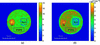Figure 4 Reconstructed results: (a) image of the effective atomic number; (b) image of the electron density. The pink dotted squares in each image are the same ROIs as in Fig. 3, chosen for the quantitative analysis of the reconstructed results.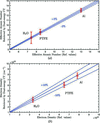Figure 5 (a) The effective atomic numbers and (b) electron densities reconstructed by dual-energy CT are plotted against the theoretical values.

Compared with the reconstruction results of the linear attenuation coefficient μ, higher Zeff precisions of the three materials are achieved as a result of the efficient Zeff retrieval method. Provided that the slope of the function f(Z) in equation (2)is steep by optimizing the low and high X-ray energies, the influence of the reconstruction precision of μ on the Zeff retrieval is not severe and high reconstruction precision of Zeff can be realized. In Fig. 2, the slope of f(Z) is a monotonically increasing function in the Z range of 5–15, hence the Zeff error bars of Al (3.10%, cf. the second column of Table 2, similarly hereinafter) and PTFE (6.56%) are much lower than that of H2O (15.94%), as shown in Fig. 5(a). Unlike the look-up table method used in Zeff retrieval, the analytical reconstruction of ρe consists of a division operation in equation (5). Therefore, the reconstruction precision of ρe is more sensitive to the reconstruction precisions of μ and Zeff, and this has been verified by the relatively high ρe reconstruction errors (>6%, cf. the second column of Table 3) of the three materials, as shown in Fig. 5(b).

 Table 2Effective atomic number Zeff: reconstructed values, reference values and their comparison.
Sample Reconstructed value Zeff C Reference value Zeff R Comparison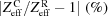Al 13.04 ± 0.40 13.00 0.27
H2O 7.41 ± 1.18 7.42 0.08
PTFE 8.57 ± 0.56 8.43 1.68
 Table 3Electron density ρe: reconstructed values, reference values and their comparison
Sample Reconstructed value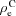(× 1023 cm−3) Reference value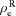(× 1023 cm−3) Comparison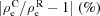Al 7.77 ± 0.50 7.83 0.82
H2O 3.33 ± 0.48 3.34 0.52
PTFE 6.08 ± 0.50 6.50 6.45

Either the effective atomic number Zeff or the electron density ρe represent a different feature of the material that is different from the conventional linear attenuation coefficient μ, hence the dual-energy CT improves the material discrimination ability of single-energy CT to a much higher dimension. Fig. 6shows a scatter diagram of Zeff and ρe of the reconstructed ROIs in Fig. 4. Note that different materials are located in different zones of the scatter diagram, based on which the above three materials can be clearly discriminated.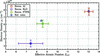Figure 6 Scatter diagram of the effective atomic number and the electron density of the reconstructed ROIs in Fig. 4.

### 4. Conclusions and outlook

The Thomson scattering X-ray source provides an excellent prospect for energy-resolved CT in laboratories and hospitals since it can generate quasi-monochromatic, energy-tunable and high-brightness X-rays with a small footprint and moderate cost. In this paper, the first proof-of-principle dual-energy CT experiment based on this type of light source has been carried out at the TTX using a standard sample, and the Zeff and ρe distribution of the sample has been reconstructed with high accuracy. The limitation of the current work is the relatively high reconstruction error of linear attenuation coefficient caused by the low SNR of our imaging system, because of which the reconstruction precisions of Zeff and ρe are not high. Improvements of the photon flux (Huang & Ruth, 1998; Eggl et al., 2016; Xu et al., 2014) and efficiency of the detection system need to be implemented in order to increase the SNR of the imaging system in the future. This initial study has demonstrated the experimental feasibility of dual-energy CT based on the Thomson light source and paved the way towards practical applications planned at the TTX, such as material testing and preclinical diagnosis of disease.

### Funding information

This work was supported by the National Natural Science Foundation of China (grant Nos. 11375097; 11435015; 11475097) and the Science Challenge Project (Grant No. TZ2018005).

### References

© International Union of Crystallography. Prior permission is not required to reproduce short quotations, tables and figures from this article, provided the original authors and source are cited. For more information, click here.JOURNAL OFSYNCHROTRONRADIATION
ISSN: 1600-5775
Volume 25| Part 6| November 2018| Pages 1797-1802